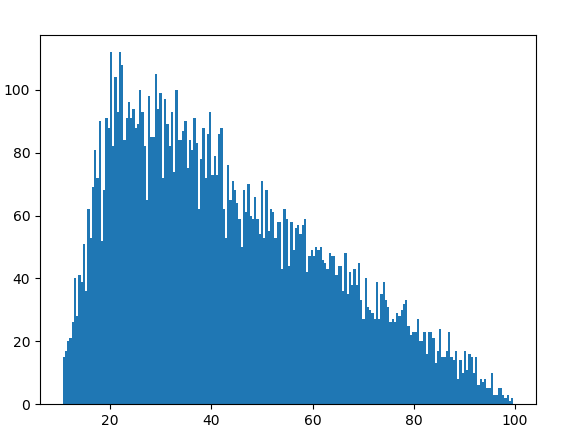GeeksforGeeks App
Open AppBrowser
Continue

# random.triangular() method in Python

`triangular()` is an inbuilt method of the `random` module. It is used to return a random floating point number within a range with a bias towards one extreme.

Syntax : random.triangular(low, high, mode)

Parameters :
low : the lower limit of the random number
high : the upper limit of the random number
mode : additional bias; low < mode < high

if the parameters are (10, 100, 20) then due to the bias, most of the random numbers generated will be closer to 10 as opposed to 100.

Returns : a random floating number

Example 1:

 `# import the random module``import` `random`` ` `# determining the values of the parameters``low ``=` `10``high ``=` `100``mode ``=` `20`` ` `# using the triangular() method``print``(random.triangular(low, high, mode))`

Output :

`22.614510550239572`

Example 2: If we generate the number multiple times we can probably identify the bias.

 `# import the random module``import` `random`` ` `# determining the values of the parameters``low ``=` `10``high ``=` `100``mode ``=` `20`` ` `# running the triangular method with the``# same parameters multiple times``for` `i ``in` `range``(``10``):``    ``print``(random.triangular(low, high, mode))`

Output :

```58.645768016894735
46.690692250503226
33.57590419190895
52.331804090351305
33.09451214875767
12.03845752596168
32.816080679206294
20.4739124559502
82.49208123077557
63.511062284733015```

Example 3: We can visualize the triangular pattern by plotting a graph.

 `# import the required libraries``import` `random``import` `matplotlib.pyplot as plt`` ` ` ` `# store the random numbers in a list``nums ``=` `[]``low ``=` `10``high ``=` `100``mode ``=` `20`` ` `for` `i ``in` `range``(``10000``):``    ``temp ``=` `random.triangular(low, high, mode)``    ``nums.append(temp)``     ` `# plotting a graph``plt.hist(nums, bins ``=` `200``)``plt.show()`

Output :My Personal Notes arrow_drop_up